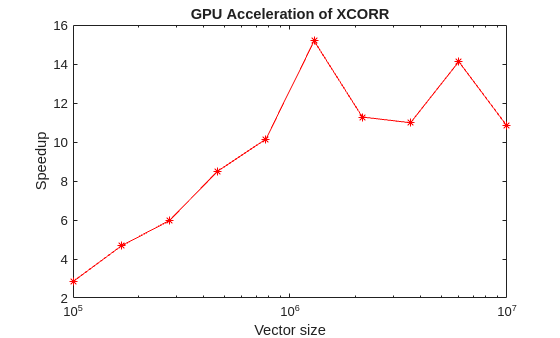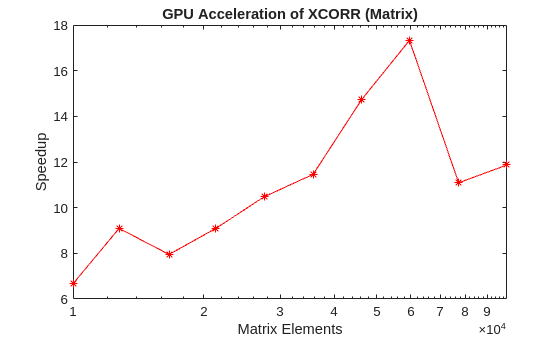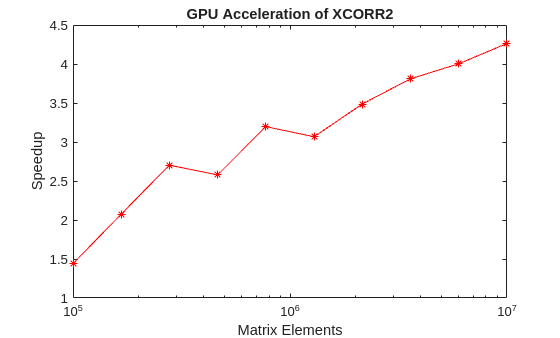# Accelerating Correlation with GPUs

This example shows how to use a GPU to accelerate cross-correlation. Many correlation problems involve large data sets and can be solved much faster using a GPU. This example requires a Parallel Computing Toolbox™ user license. Refer to GPU Support by Release (Parallel Computing Toolbox) to see what GPUs are supported.

### Introduction

Start by learning some basic information about the GPU in your machine. To access the GPU, use the Parallel Computing Toolbox.

```fprintf('Benchmarking GPU-accelerated Cross-Correlation.\n'); if ~(parallel.gpu.GPUDevice.isAvailable) fprintf(['\n\t**GPU not available. Stopping.**\n']); return; else dev = gpuDevice; fprintf(... 'GPU detected (%s, %d multiprocessors, Compute Capability %s)',... dev.Name, dev.MultiprocessorCount, dev.ComputeCapability); end ```
```Benchmarking GPU-accelerated Cross-Correlation. GPU detected (TITAN Xp, 30 multiprocessors, Compute Capability 6.1)```

### Benchmarking Functions

Because code written for the CPU can be ported to run on the GPU, a single function can be used to benchmark both the CPU and GPU. However, because code on the GPU executes asynchronously from the CPU, special precaution should be taken when measuring performance. Before measuring the time taken to execute a function, ensure that all GPU processing has finished by executing the 'wait' method on the device. This extra call will have no effect on the CPU performance.

This example benchmarks three different types of cross-correlation.

### Benchmark Simple Cross-Correlation

For the first case, two vectors of equal size are cross-correlated using the syntax `xcorr(u,v)`. The ratio of CPU execution time to GPU execution time is plotted against the size of the vectors.

```fprintf('\n\n *** Benchmarking vector-vector cross-correlation*** \n\n'); fprintf('Benchmarking function :\n'); type('benchXcorrVec'); fprintf('\n\n'); sizes = [2000 1e4 1e5 5e5 1e6]; tc = zeros(1,numel(sizes)); tg = zeros(1,numel(sizes)); numruns = 10; for s=1:numel(sizes); fprintf('Running xcorr of %d elements...\n', sizes(s)); delchar = repmat('\b', 1,numruns); a = rand(sizes(s),1); b = rand(sizes(s),1); tc(s) = benchXcorrVec(a, b, numruns); fprintf([delchar '\t\tCPU time : %.2f ms\n'], 1000*tc(s)); tg(s) = benchXcorrVec(gpuArray(a), gpuArray(b), numruns); fprintf([delchar '\t\tGPU time : %.2f ms\n'], 1000*tg(s)); end %Plot the results fig = figure; ax = axes('parent', fig); semilogx(ax, sizes, tc./tg, 'r*-'); ylabel(ax, 'Speedup'); xlabel(ax, 'Vector size'); title(ax, 'GPU Acceleration of XCORR'); drawnow; ```
``` *** Benchmarking vector-vector cross-correlation*** Benchmarking function : function t = benchXcorrVec(u,v, numruns) %Used to benchmark xcorr with vector inputs on the CPU and GPU. % Copyright 2012 The MathWorks, Inc. timevec = zeros(1,numruns); gdev = gpuDevice; for ii=1:numruns ts = tic; o = xcorr(u,v); %#ok<NASGU> wait(gdev) timevec(ii) = toc(ts); fprintf('.'); end t = min(timevec); end Running xcorr of 2000 elements... CPU time : 0.21 ms GPU time : 4.26 ms Running xcorr of 10000 elements... CPU time : 1.03 ms GPU time : 4.37 ms Running xcorr of 100000 elements... CPU time : 14.04 ms GPU time : 6.28 ms Running xcorr of 500000 elements... CPU time : 55.98 ms GPU time : 16.09 ms Running xcorr of 1000000 elements... CPU time : 169.00 ms GPU time : 25.60 ms ```### Benchmarking Matrix Column Cross-Correlation

For the second case, the columns of a matrix A are pairwise cross-correlated to produce a large matrix output of all correlations using the syntax xcorr(A). The ratio of CPU execution time to GPU execution time is plotted against the size of the matrix A.

```fprintf('\n\n *** Benchmarking matrix column cross-correlation*** \n\n'); fprintf('Benchmarking function :\n'); type('benchXcorrMatrix'); fprintf('\n\n'); sizes = floor(linspace(0,100, 11)); sizes(1) = []; tc = zeros(1,numel(sizes)); tg = zeros(1,numel(sizes)); numruns = 10; for s=1:numel(sizes); fprintf('Running xcorr (matrix) of a %d x %d matrix...\n', sizes(s), sizes(s)); delchar = repmat('\b', 1,numruns); a = rand(sizes(s)); tc(s) = benchXcorrMatrix(a, numruns); fprintf([delchar '\t\tCPU time : %.2f ms\n'], 1000*tc(s)); tg(s) = benchXcorrMatrix(gpuArray(a), numruns); fprintf([delchar '\t\tGPU time : %.2f ms\n'], 1000*tg(s)); end %Plot the results fig = figure; ax = axes('parent', fig); plot(ax, sizes.^2, tc./tg, 'r*-'); ylabel(ax, 'Speedup'); xlabel(ax, 'Matrix Elements'); title(ax, 'GPU Acceleration of XCORR (Matrix)'); drawnow; ```
``` *** Benchmarking matrix column cross-correlation*** Benchmarking function : function t = benchXcorrMatrix(A, numruns) %Used to benchmark xcorr with Matrix input on CPU and GPU. % Copyright 2012 The MathWorks, Inc. timevec = zeros(1,numruns); gdev = gpuDevice; for ii=1:numruns, ts = tic; o = xcorr(A); %#ok<NASGU> wait(gdev) timevec(ii) = toc(ts); fprintf('.'); end t = min(timevec); end Running xcorr (matrix) of a 10 x 10 matrix... CPU time : 0.18 ms GPU time : 5.00 ms Running xcorr (matrix) of a 20 x 20 matrix... CPU time : 0.48 ms GPU time : 4.83 ms Running xcorr (matrix) of a 30 x 30 matrix... CPU time : 0.85 ms GPU time : 4.84 ms Running xcorr (matrix) of a 40 x 40 matrix... CPU time : 3.38 ms GPU time : 5.57 ms Running xcorr (matrix) of a 50 x 50 matrix... CPU time : 5.60 ms GPU time : 5.22 ms Running xcorr (matrix) of a 60 x 60 matrix... CPU time : 8.49 ms GPU time : 5.39 ms Running xcorr (matrix) of a 70 x 70 matrix... CPU time : 20.43 ms GPU time : 5.92 ms Running xcorr (matrix) of a 80 x 80 matrix... CPU time : 26.79 ms GPU time : 6.24 ms Running xcorr (matrix) of a 90 x 90 matrix... CPU time : 40.04 ms GPU time : 6.89 ms Running xcorr (matrix) of a 100 x 100 matrix... CPU time : 49.69 ms GPU time : 7.32 ms ```### Benchmarking Two-Dimensional Cross-Correlation

For the final case, two matrices, X and Y, are cross correlated using xcorr2(X,Y). X is fixed in size while Y is allowed to vary. The speedup is plotted against the size of the second matrix.

```fprintf('\n\n *** Benchmarking 2-D cross-correlation*** \n\n'); fprintf('Benchmarking function :\n'); type('benchXcorr2'); fprintf('\n\n'); sizes = [100, 200, 500, 1000, 1500, 2000]; tc = zeros(1,numel(sizes)); tg = zeros(1,numel(sizes)); numruns = 4; a = rand(100); for s=1:numel(sizes); fprintf('Running xcorr2 of a 100x100 matrix and %d x %d matrix...\n', sizes(s), sizes(s)); delchar = repmat('\b', 1,numruns); b = rand(sizes(s)); tc(s) = benchXcorr2(a, b, numruns); fprintf([delchar '\t\tCPU time : %.2f ms\n'], 1000*tc(s)); tg(s) = benchXcorr2(gpuArray(a), gpuArray(b), numruns); fprintf([delchar '\t\tGPU time : %.2f ms\n'], 1000*tg(s)); end %Plot the results fig = figure; ax =axes('parent', fig); semilogx(ax, sizes.^2, tc./tg, 'r*-'); ylabel(ax, 'Speedup'); xlabel(ax, 'Matrix Elements'); title(ax, 'GPU Acceleration of XCORR2'); drawnow; fprintf('\n\nBenchmarking completed.\n\n'); ```
``` *** Benchmarking 2-D cross-correlation*** Benchmarking function : function t = benchXcorr2(X, Y, numruns) %Used to benchmark xcorr2 on the CPU and GPU. % Copyright 2012 The MathWorks, Inc. timevec = zeros(1,numruns); gdev = gpuDevice; for ii=1:numruns, ts = tic; o = xcorr2(X,Y); %#ok<NASGU> wait(gdev) timevec(ii) = toc(ts); fprintf('.'); end t = min(timevec); end Running xcorr2 of a 100x100 matrix and 100 x 100 matrix... CPU time : 20.35 ms GPU time : 6.96 ms Running xcorr2 of a 100x100 matrix and 200 x 200 matrix... CPU time : 42.87 ms GPU time : 11.72 ms Running xcorr2 of a 100x100 matrix and 500 x 500 matrix... CPU time : 125.23 ms GPU time : 39.67 ms Running xcorr2 of a 100x100 matrix and 1000 x 1000 matrix... CPU time : 386.59 ms GPU time : 88.46 ms Running xcorr2 of a 100x100 matrix and 1500 x 1500 matrix... CPU time : 788.38 ms GPU time : 165.04 ms Running xcorr2 of a 100x100 matrix and 2000 x 2000 matrix... CPU time : 1523.05 ms GPU time : 279.55 ms Benchmarking completed. ```### Other GPU Accelerated Signal Processing Functions

There are several other signal processing functions that can be run on the GPU. These functions include fft, ifft, conv, filter, fftfilt, and more. In some cases, you can achieve large acceleration relative to the CPU. For a full list of GPU accelerated signal processing functions, see the GPU Algorithm Acceleration section in the Signal Processing Toolbox™ documentation.